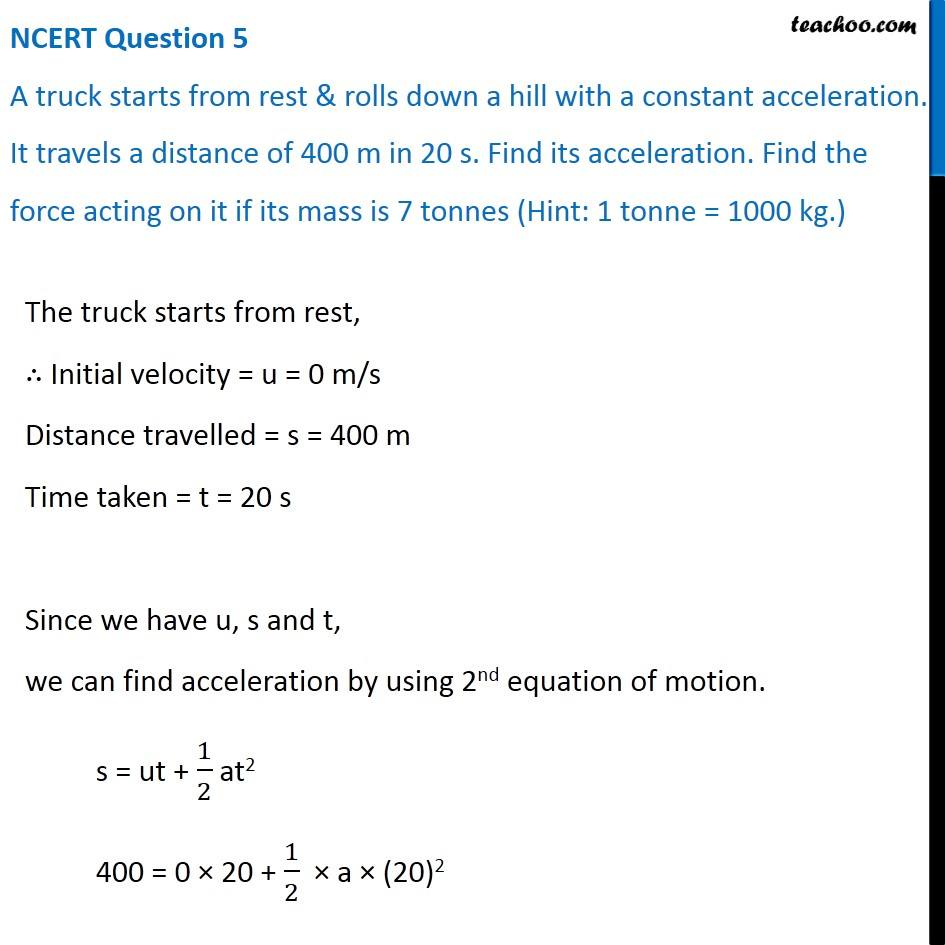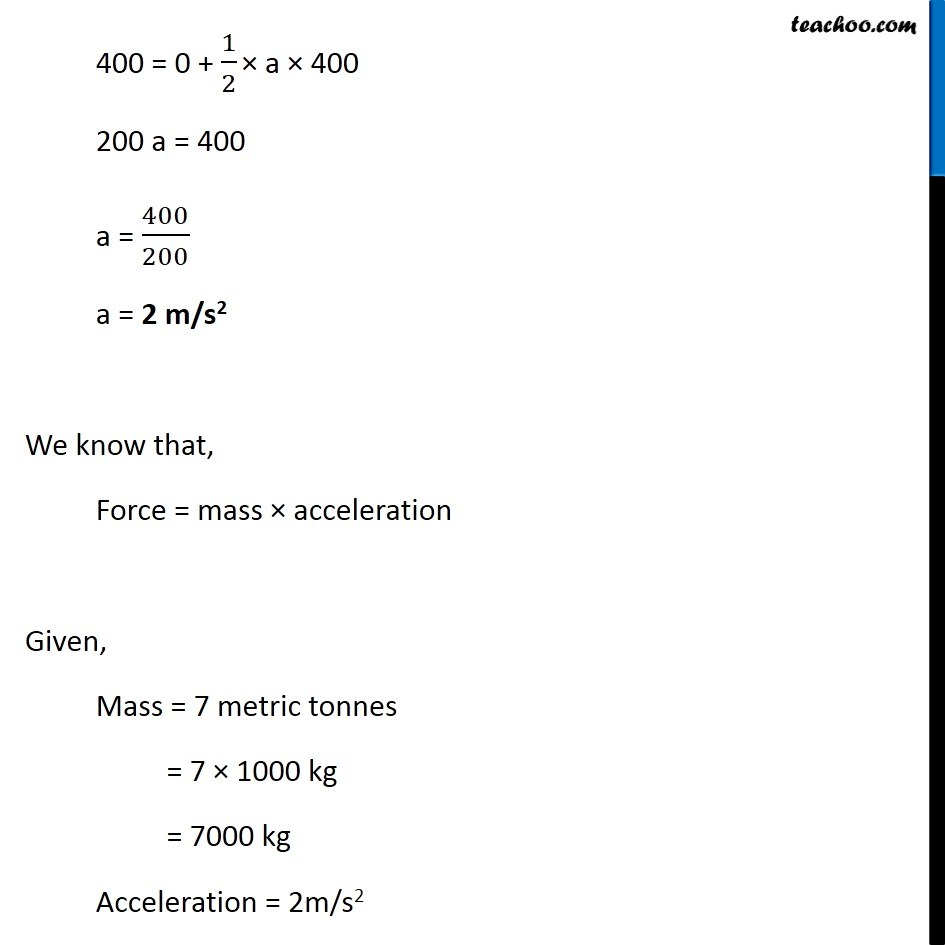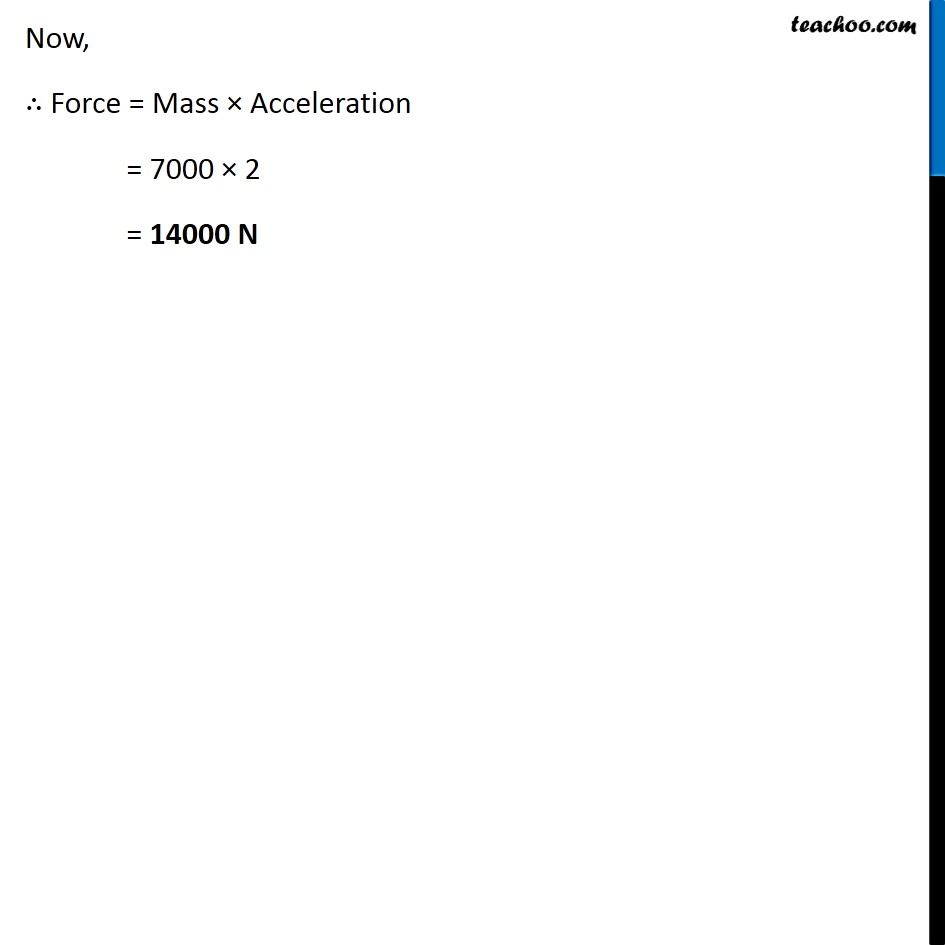NCERT Questions

Class 9
Chapter 9 Class 9 - Force and Laws Of MotionLearn in your speed, with individual attention - Teachoo Maths 1-on-1 Class

### Transcript

NCERT Question 5 A truck starts from rest & rolls down a hill with a constant acceleration. It travels a distance of 400 m in 20 s. Find its acceleration. Find the force acting on it if its mass is 7 tonnes (Hint: 1 tonne = 1000 kg.) The truck starts from rest, ∴ Initial velocity = u = 0 m/s Distance travelled = s = 400 m Time taken = t = 20 s Since we have u, s and t, we can find acceleration by using 2nd equation of motion. s = ut + 1/2 at2 400 = 0 × 20 + 1/2 × a × (20)2 400 = 0 + 1/2 × a × 400 200 a = 400 a = 400/200 a = 2 m/s2 We know that, Force = mass × acceleration Given, Mass = 7 metric tonnes = 7 × 1000 kg = 7000 kg Acceleration = 2m/s2 Now, ∴ Force = Mass × Acceleration = 7000 × 2 = 14000 N